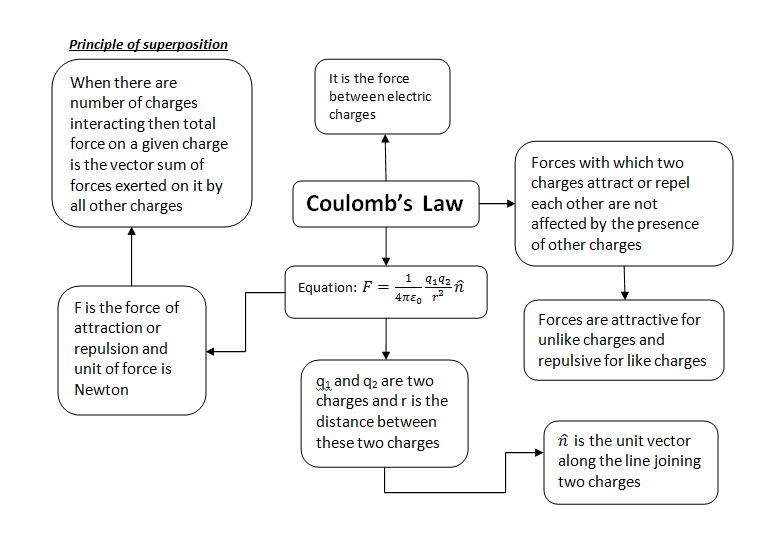Home » General » How to solve electric force and field problems

# How to solve electric force and field problems

[latexpage]

## Solve electric force and field problems easily

Electric Force problems can be bit tricky to solve. This post is all about how you can easily solve electric force and electric field problems. At a glance problems of physics can seem to be scary and overwhelming for some students but do not worry all you need to do is your willingness to solve problem. So first of all you need to read your problem carefully and then start using following protocol to solve your problems. The procedure I am going to write is for calculating electric forces  but you can use the same procedure to calculate an electric field by simply replacing the charge of interest, q, with a convenient test charge and dividing by the test charge at the end. So, to calculate the force,

1. Draw a diagram of the charges in the problem.
2. Identify the charge of interest, q, and circle it.
3. Convert all units to SI, with charges in coulombs and distances in meters, so as to be consistent with the SI value of the Coulomb constant k e .
4. Apply Coulomb’s law. For each charge Q , find the electric force on the charge of interest, q. The magnitude of the force can be found using Coulomb’s law. The vector direction of the electric force is along the line of the two charges, directed away from Q if the charges have the same sign, toward Q if the charges have the opposite sign. Find the angle $\theta$ this vector makes with the positive x -axis. The x-component of the electric force exerted by Q on q will be $F cos \theta$ , and the
y-component will be $F sin \theta$ .
5. Sum all the x-components, getting the x-component of the resultant electric force.
6. Sum all the y-components, getting the y-component of the resultant electric force.
7. Use the Pythagorean theorem and trigonometry to find the magnitude and direction of the resultant force if desired.

Following is a concept map of understanding Coulomb’s Law. It might prove helpful for memorizing concepts at a glanceThis site uses Akismet to reduce spam. Learn how your comment data is processed.